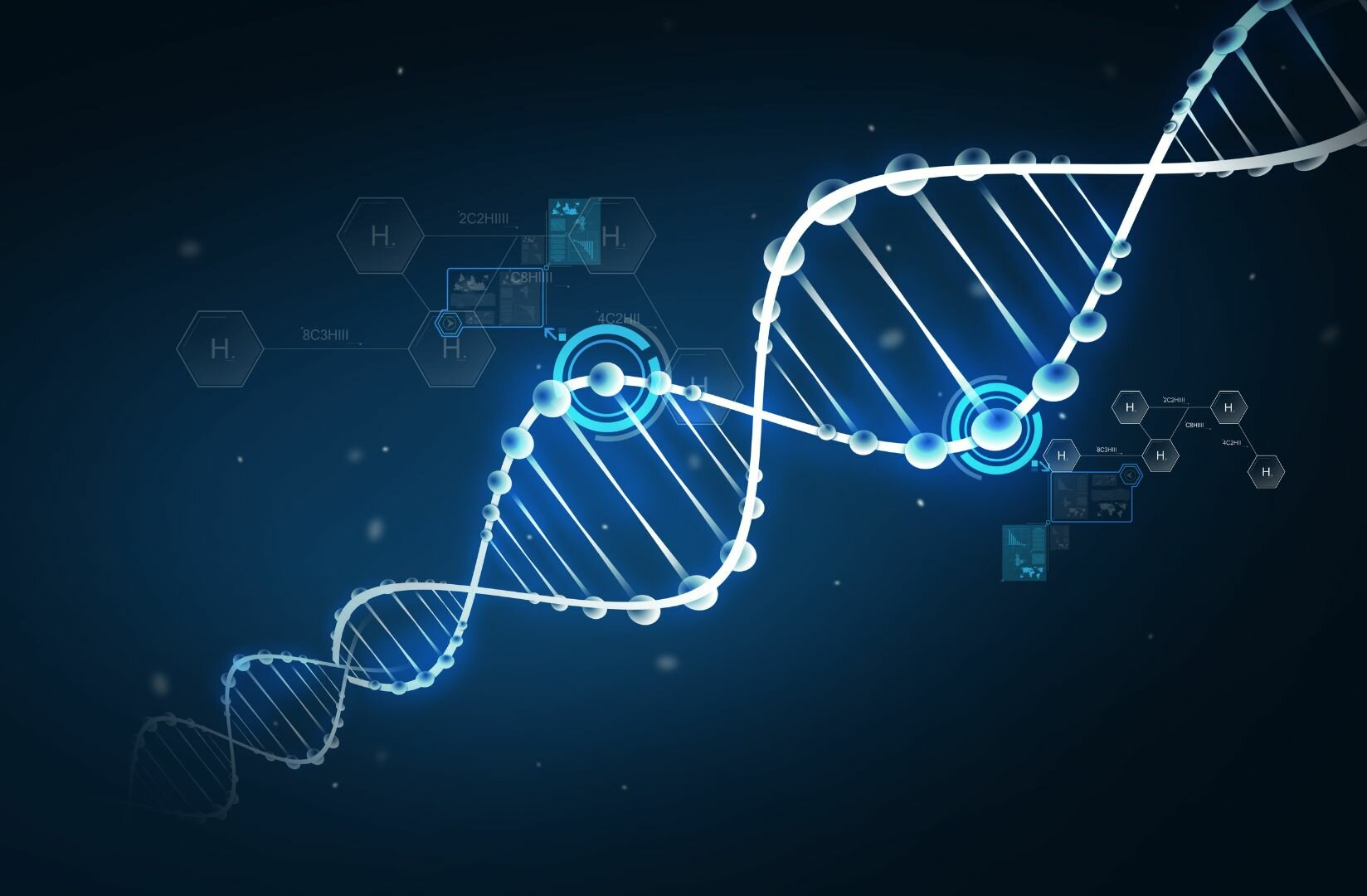Need help?

# The Molar Mass of H2

Molar mass is an essential part of any high school curriculum. Whether you’re measuring how much sodium, chromium or cobalt to add to an experiment, an element’s molar mass is essential in calculating exactly how much of a substance to add to a scientific experiment. In this article, you’ll find all the facts about the molar mass of H2, including how to calculate it and why it’s so important in science classes.

#### What is Molar Mass?

Every scientific experiment requires precise measurements of substances. If you want to come to valid scientific conclusions, the precise weight of substances like chromium, nickel or iron must be measured with high accuracy. This is exactly why molar mass is so important.

Here’s an example: say you need 6 moles of pure aluminum for an experiment. You already know aluminum has a molar mass of 26.923 g/mol. All you need to do to find out how much to weigh is simply multiply 26.923 by 6. You’ll need 161.538g of aluminum.

#### What’s the Molar Mass of H2?

The molar mass of H2 is 2.01568 g/mole [quote]. Because hydrogen gas is formed of two hydrogen (H) molecules bound together (H2), the molar mass can be calculated by multiplying the atomic weight of hydrogen (1.00784 atomic mass units) by 2. [quote] 1.00784 x 2 = 2.01568 grams.#### Hydrogen: A General Overview

Hydrogen has no smell, color, or taste. It’s a noble gas, but is known for being extremely flammable.

Hydrogen is also an extremely ‘light’ element. When an atom has more protons in its nucleus, its atomic number will be higher, and it will therefore become heavier. Hydrogen has an atomic number of 1 (meaning its nucleus only consists of one proton), making it the lightest element in the periodic table.

Another thing hydrogen is known for is its reactivity. In fact, atomic hydrogen (H) is so reactive that two hydrogen atoms naturally combine together to form hydrogen gas (H2).

Because of its reactivity, molecular hydrogen (H2) also reacts with lots of elements and compounds. Why doesn’t hydrogen react with everything, you might ask? At room temperature, these reactions take place at such a slow rate that they’re almost negligible. It is because of this inertness that the H2 molecule is considered very stable at room temperature.

#### Uses of Hydrogen in Education

One of the newest ways schools are teaching kids about the fun and varied uses of hydrogen is by demonstrating how it can be used to power fuel cells in cars. If you’re interested in seeing how hydrogen can be used to power fuel-cell electric cars, the Horizon Hydrogen Grand Prix (H2GP) provides a unique introduction to the production, storage, and end-use of green hydrogen. This allows you to understand how what people call hydrogen’s complete value chain.

Interested in learning more about how hydrogen can be produced, stored and used? STEM kits are a great way of understanding the real-life processes by which hydrogen can be produced from solar energy, stored in thumb-size storage containers, or used to power a variety of motors in cars